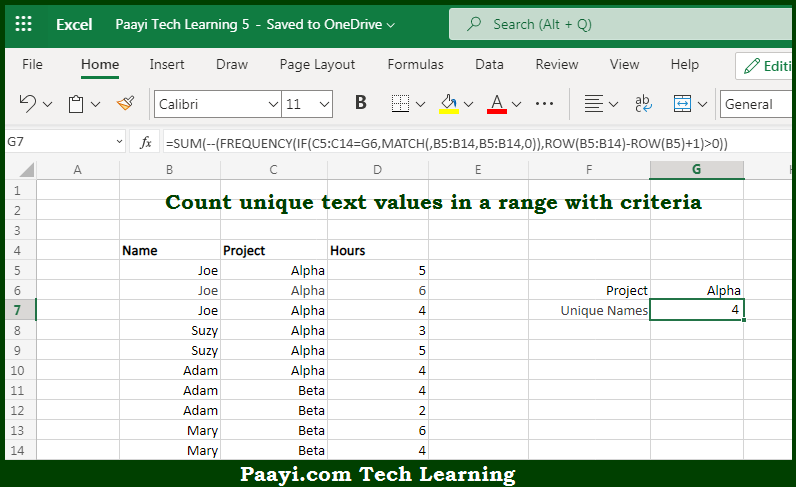# Learn How to COUNT Unique Text Values With a Criteria in Microsoft Excel

Written by | 0 Comments | 660 Views

In this article, you will learn how to COUNT various things in Microsoft Excel using a single or combination of functions and its purpose. You will also get to know how to COUNT Unique Text Values With Criteria and see the generic formula.

COUNT Unique Text Values With a Criteria in Microsoft Excel

The main purpose of this formula is to count unique text values in a range with criteria. Here we will learn how to count unique text values in the given range with criteria in Microsoft Excel. That implies, with the help of a formula based on the FREQUENCY, and MATCH function you can able to count unique text values in a range with criteria. So, with the help of this formula, you can able to count unique text values in the given range with criteria.

General Formula to COUNT Unique Text Values With a Criteria

{=SUM(--(FREQUENCY(IF(criteria,MATCH(vals,vals,0)),ROW(vals)-ROW(vals.first)+1)>0))}

The Explanation for the COUNT Unique Text Values With a CriteriaSo we know that with the help of the given formula above you can able to count unique text values in a range with criteria. Here we will learn how to count unique text values in the given range with criteria in Microsoft Excel. As we know that usually, the formula provided here is more complicated than a similar formula that uses FREQUENCY to count unique numeric values because FREQUENCY doesn't work with non-numeric values. As a result, a large part of the formula simply transforms the non-numeric data into numeric data that FREQUENCY can handle. Working from the inside-out, the MATCH function is used to get the position of each item that appears in the data. So, with the help of this formula, you can able to count unique text values in a range with criteria.